# AttenuatorsAten, the Egyptian Sun God that you attenuate with SPF-45!

Here's a clickable index our material on attenuators:

Click here to view a page on amplifier temperature compensation (new for March 2022)

Carrottenuator

Reflection attenuators

Search for RF attenuators on EverythingRF.com

Introduction to attenuators

Fixed attenuators

Clifton Quan's patented attenuator

Temperature-compensating fixed attenuators

Power dissipation in attenuators

Attenuator math

Switchable attenuators

Switched-network attenuators

Switched-element attenuators

Digital attenuators (stepped attenuators)

RMS amplitude and phase of switchable attenuators

Variable attenuators

Ray Waugh's PIN diode attenuator

Gain equalizers

Parabolic equalizers

Parabolic equalizer example 2 (new for April 2022)

## Introduction to attenuators

Attenuators are passive resistive elements that do the opposite of amplifiers, they kill gain. Why would you want to do that? Suppose your design specification calls for 10 dB gain, with a 1.2:1 maximum VSWR. You search the amplifier vendors, and locate an amplifier in your frequency band, but it has 14.5 dB gain and a lousy 2.5:1 match on the input. By adding an attenuator to the input, you can bring the gain down to 10 dB, and you will be improving the input match. Two things to consider when you play this game: don’t add an attenuator to an amplifier’s input if you are concerned with the amplifier’s noise figure, every dB of attenuation you put on the input will raise the noise figure by the same amount. Similarly, don’t add an attenuator to a power amplifier’s output without considering what it will do to your output power, or what the RF output power of the power amp might do to your attenuator.

There are five common attenuator topologies used in microwave circuits, the tee, the pi, the bridged tee, the reflection attenuator and the balanced attenuator. The tee, pi and bridged tee each require two different resistor values, while the reflection and balanced attenuators need only a matched pair of resistors. This allows both the reflection and balanced topologies to be used as variable attenuators with a single control voltage or control current. There are two variations of the reflection attenuator, depending on whether the terminations R1 are less than or greater than the system characteristic impedance Z0.

When would you use a tee versus a pi versus a bridged tee? Here's some examples. When you are designing a fixed-value 3-dB attenuator on a thin film circuit, with a sheet resistivity fixed at 100 ohms per square, the 8.5 ohm value of R1 for a tee might be a little hard to accurately etch, and the pi might be a better choice. On the other hand, if your sheet resistivity was 10 ohms per square, you'd need 29 squares to create R1 for the pi, and that might prove to be too inductive to work at high frequency. With thick film circuits, you can take your pick because there are different resistivity values available.

The bridged tee can be thought of as a modified pi network. The attraction to the bridged tee comes when you are making a variable attenuator, with PIN diodes or FETs. Here are two reasons you might consider it over the pi. First, it only needs two variable resistors (pi and tee need three). Second, the bridged tee uses the full range of resistor values, from zero to infinity, for both R1 and R2. For the pi attenuator, R1 never goes below Z0 (50 ohms) so some of the diodes' useful resistance range is wasted. Finally, the bridged tee has a tendency to match itself to Z0 at high attenuation values, because of its two fixed resistors. In practice, the pi may give you higher attenuation range. The resistor R2 can be a "sneak path" in the bridged tee because the diode (or FET) never reaches zero ohms.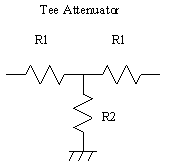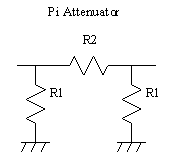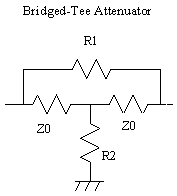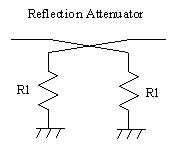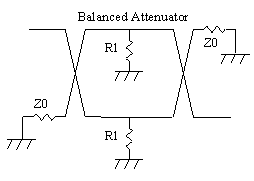The table below provides equations for solving for the attenuator resistive elements. It is incomplete; did you know that the tee and pi attenuators alone can have twelve forms of these equations, each? Think about this: there are four variables (Z0, R1, R2, and dB) and only two must be chosen to lock in the other two.

Attenuator equations

Configuration

R2 vs. R1

R1 vs. Attenuation

R2 vs. Attenuation

Tee Attenuator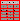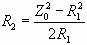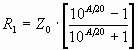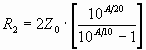Pi Attenuator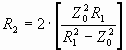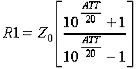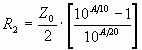Bridged Tee Attenuator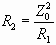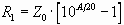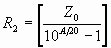Reflection Attenuator,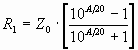Reflection Attenuator,Balanced Attenuator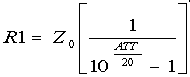Click on the calculator icon () to check out our calculator where you'll be able to enter your desired attenuation parameters and we will calculate the resistors for you.

If you use the above equations to calculate R1 and R2, be sure to observe whether "ATT" is divided by 10 or 20. For those of you too busy or lazy to calculate your own resistor values, the table below should be useful (Z0 is assumed to be 50 ohms, but you can scale the values if necessary).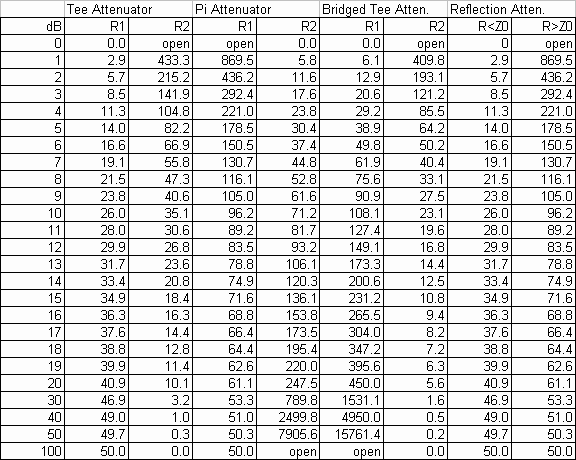Turns out for the tee, pi and bridged tee attenuators, there are four parameters: R1, R2, Z0 and dB. If you pick any one of them, the other two can be solved. Why would you want to pick dB and Z0 instead of R1 and R2? Trust us, there are situations where that would be useful. Of course you can iterative solve any of the equations to get the answers you seek, but why not come up with elegant, closed form equations?

There are twelve possible equations for each attenuator type. We have completed the solutions for the tee, and much of the pi attenuator. Below we post the solutions. If someone can help us finish the missing ones we'd appreciate that! You can cut and paste them into Excel and verify that they work, or ask us nicely for a spreadsheet that already has them in it!

### Tee attenuator - twelve equations

 Pick R1, R2: dB=20*LOG(SQRT(R1^2+2*R1*R2)+R1)-20*LOG(SQRT(R1^2+2*R1*R2)-R1) Z0=SQRT(R1^2+2*R1*R2) Pick R1, Z0: R2=(Z0^2-R1^2)/2/R1 dB=20*LOG(Z0+R1)-20*LOG(Z0-R1) Pick dB, R1: R2=2*R1*(10^(dB/20)+1)*(10^(dB/20))/(10^(dB/10)-1)/(10^(dB/20)-1) Z0=R1*(10^(dB/20)+1)/(10^(dB/20)-1) Pick R2, Z0: R1=SQRT(R2^2+Z0^2)-R2 dB=20*LOG(Z0+SQRT(Z0^2+R2^2))-20*LOG(R2) Pick dB, R2: R1=2*R2/((10^(dB/20)+1)^2/(10^(dB/20)-1)^2-1) Z0=(R2/2)*(10^(dB/10)-1)/10^(dB/20) Pick dB, Z0: R1=Z0*(10^(dB/20)-1)/(10^(dB/20)+1) R2=2*Z0*10^(dB/20)/(10^(dB/10)-1)

### Pi attenuator - twelve equations

 Pick R1, R2: Coming soon! Pick R1, Z0: R2=2*Z0^2*R1/(R1^2-Z0^2) dB=20*LOG((R1+Z0)/(R1-Z0)) Pick dB, R1: Coming soon! Pick R2, Z0: R1=(Z0^2+Z0*SQRT(Z0^2+R2^2))/R2 dB=20*LOG((R2/Z0)+SQRT((R2/Z0)^2+1)) Pick dB, R2: Coming soon! Pick dB, Z0: R1=Z0*(10^(dB/20)+1)/(10^(dB/20)-1) R2=(Z0/2)*(10^(dB/10)-1)/10^(dB/20)

## Fixed attenuators

There are many commercially available fixed attenuators, including chip, coaxial, and waveguide implementations. Two considerations you will need to consider are frequency of operation, and power handling.

Chip attenuators can dissipate hundreds of milliwatts up to a few watts. The higher power units will be realized on thermally conductive substrates such as beryllium oxide and aluminum nitride.

If you are designing your own attenuator, the resistive elements can be realized with either thick film or thin film processes. While the thick film process can use different "inks" for different resistors, providing different ohm/square values, the thin film process uses one sheet resistance for all resistors. Having different inks solves the problem of making compact resistors of two radically different values, for instance, a 1 dB tee attenuator would require an R1 of 2.9 ohms and an R2 value of 433 ohms. Large value thin film resistors can be made compact by using a meandered layout. In both thick and thin film processes, the "as-fired" tolerance is usually no better than 15%. Both types of resistors can be trimmed with a laser to tolerances of less than 1% (see also thin film resistors).

There are two opposing concerns in microwave attenuator design: the high frequency of operation requires that resistive elements be kept small to be considered "lumped elements" (i.e. less than 1/10 wavelength), and power handling requires that resistors be made as large as possible to dissipate heat. The heat issue can be dealt with for small resistors if attention is paid to the material properties of the resistors’ substrates and attachment methods, i.e. beryllium oxide for a substrate instead of alumina and solder attachment instead of conductive epoxy (see also material properties and heat dissipation).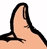Rule of thumb: a chip attenuator is good for at least 1/16 watt if it is mounted to a circuit card such as Duroid or FR-4, 1/4 watt if mounted to metal with conductive epoxy, and 1/2 watt if it is attached with solder to a metal heatsink.

## Temperature compensating fixed attenuators

One very useful type of attenuator is a temperature compensating attenuator. These devices are used to offset the gain versus temperature effect of active stages such as amplifiers. Typically the temperature compensating attenuator has a positive attenuation/temperature coefficient, i.e. the loss gets reduced with increasing temperature, the opposite of what happens to an amplifier. There is only one supplier for temp-comp attenuators that we know of.

Author : Unknown Editor